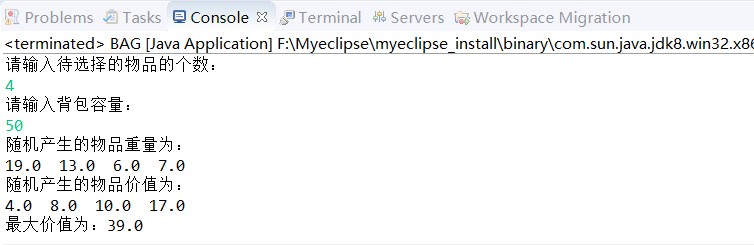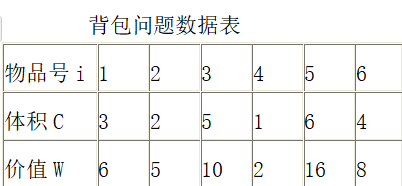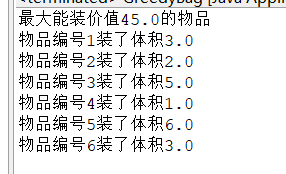• 贪心算法解决01背包问题
2021-12-27 20:32:44
/*贪心算法解决部分背包问题
w：记录各个商品的总重量
p：记录各个商品的总价值
n: 商品数量
result：记录各个商品装入背包的比例
W：背包的容量
*/

void Swap(float* x, float* y)
{
int tmp = 0;
tmp = *x;
*x = *y;
*y = tmp;
}

//根据收益率，对记录的商品进行从大到小排序
void Sort(float* w, float* p,int n)
{
int i, j;

float* v=(float*)malloc(sizeof(float)*n);//收益率
if (v == NULL)
{
printf("失败");
exit(-1);
}
for (i = 0; i < n; i++)
{
v[i] = p[i] / w[i];
}
for (i = 0; i < n; i++)
{
for (j = i + 1; j < n; j++)
{
if (v[i] < v[j])
{
Swap(&v[i], &v[j]);
Swap(&w[i], &w[j]);
Swap(&p[i], &p[j]);
}
}
}

}

void FractionalKnapsack(float* w, float* p, float* result, float W,int n)
{
int i = 0;
float tmp = 0;
Sort(w, p, n);
while (W > 0)
{
tmp = W > w[i] ? w[i] : W;
result[i] = tmp / w[i];
W -= tmp;
i++;

}
}贪心算法
更多相关内容
• 问题描述如下： 给定n种物品和一个背包。...经典的背包问题，这次选用贪心算法解决 代码如下： package TXSF; import java.util.Scanner; public class BAG { public static void main(String a...

问题描述如下：

给定n种物品和一个背包。物品i的重量是Wi，其价值为Vi，背包的容量为C。应如何选择装入背包的物品，使得装入背包中物品的总价值最大?

经典的背包问题，这次选用贪心算法来解决

代码如下：

package TXSF;

import java.util.Scanner;

public class BAG {
public static void main(String args[]){
int n,i,j;
double C;
System.out.println("请输入待选择的物品的个数：");
Scanner scanner = new Scanner(System.in);
n = scanner.nextInt();
System.out.println("请输入背包容量：");
C = scanner.nextDouble();
double[] a = new double[n];    //物品重量数组
double[] b = new double[n];    //物品价值数组
double[] x = new double[n];
for(i = 0; i < n; i++){
a[i] = Math.floor(Math.random()*20);
}
for(i = 0; i < n; i++){
b[i] = Math.floor(Math.random()*20);
}
System.out.println("随机产生的物品重量为：");
for(i = 0; i < n; i++){
System.out.print(a[i]+"  ");
}
System.out.println();
System.out.println("随机产生的物品价值为：");
for(i = 0; i < n; i++){
System.out.print(b[i]+"  ");
}
System.out.println();
double value = Knapsack(a,b,x,C,n);
System.out.println("最大价值为："+value);
}
static void sort(double[] a, double[] b, int n){
double[] c = new double[n];
for(int i = 1; i < n; i++){
c[i] = b[i]/a[i];
}
for(int i = 1; i < n; i++){
for(int j = 1; j < n-i; j++){
if(c[j] < c[j+1]){

double temp=c[j];
c[j]=c[j+1];
c[j+1]=temp;

double temp2=a[j];
a[j]=a[j+1];
a[j+1]=temp2;

double temp3=b[j];
b[j]=b[j+1];
b[j+1]=temp3;
}
}
}
}
static double Knapsack(double[] a, double[] b, double[] x, double C, int n){
sort(a,b,n);
int i;
double total = 0;
for (i = 0; i < n; i++) {
if (a[i] <= C){//如果背包剩余的容量大于等于第i个物体的重量
x[i] = 1;   //把第i个物体整个装进背包
C = C - a[i];  //背包的剩余容量减少了第i个物体的重量
}else {
break;  //退出循环
}
}
if (i < n){//判断是否第n个物体整个装进去背包里了,如果i<=n表示否定
x[i] = C/a[i];
}
for(i = 0; i < n; i++){
total = total+x[i]*b[i];
}
}
}



运行截图如下：如代码有误，欢迎指出。

展开全文• 主要介绍了JS基于贪心算法解决背包问题,简单说明了贪心算法的概念、原理,并结合具体实例形式分析了JS使用贪心算法解决部分背包问题的具体操作技巧,需要的朋友可以参考下
• 本文实例讲述了Python基于贪心算法解决背包问题。分享给大家供大家参考，具体如下： 贪心算法（又称贪婪算法）是指，在对问题求解时，总是做出在当前看来是最好的选择。也就是说，不从整体最优上加以考虑，他所做出...
• 题目： 用贪心算法实现背包问题的求解。背包容量为20；最优解为装入背包的物品价值总和最大。...public class GreedyBag {// 贪心算法解决背包问题是可以部分装载的问题，不是0-1 static float maxV = ...

## 题目：

用贪心算法实现背包问题的求解。背包容量为20；最优解为装入背包的物品价值总和最大。## 基本思想：

•  计算所有物品的性价比
• 按物品性价比从高到低装入，只有当高一级的性价比物品全部装入后，才会装入下一级的性价比物品。
• 装到最后无法全部装入该物品时进行部分装入

## 代码结果：## 代码如下：

package algorism4;

public class GreedyBag {// 贪心算法解决的背包问题是可以部分装载的问题，不是0-1
static float maxV = 20;// 最大容量
float V;// 体积
float worth;// 价值
int i;// 物品编号
float cost;// 性价比,单位体积价值
static GreedyBag[] thing = new GreedyBag;// 根据实际情况调整
static float[] x = new float[thing.length];// 物品i对应的数量在下标i-1

public GreedyBag(int num, float V, float w) {// 物品信息初始化
i = num;
worth = w;
this.V = V;
cost = w / V;
thing[i - 1] = this;// 添加进数组
}

public static void sort() {// 初始化满了再用的冒泡排序,性价比最小的沉底
float smaller;// 存储更小的价值比
for (int i = 0; i < thing.length; i++) {
for (int j = 0; j < thing.length - i - 1; j++) {
smaller = thing[j].cost;
if (smaller < thing[j + 1].cost) {// 后面更大就交换
GreedyBag bigger = thing[j + 1];
thing[j + 1] = thing[j];
thing[j] = bigger;
}
}
}
}

public static float knapsack() {
float maxValue = 0;
float v = 0;// 已装载体积
int i;
for (int j = 0; j < x.length; j++)
x[j] = 0;// 物品的装载初始化为0
for (i = 0; i < thing.length; i++) {
if (v + thing[i].V > maxV)
break;// 下标i的物品没法全部装下
x[thing[i].i - 1] = thing[i].V;// 性价比高的全部装下
v += thing[i].V;
maxValue += thing[i].worth;
}
if (i < thing.length) {// 由break跳出的，部分装载
float elseV = maxV - v;
x[thing[i].i - 1] = elseV;// 部分装载
v = maxV;
maxValue += elseV / thing[i].V * thing[i].worth;
}
return maxValue;
}

public static void printX() {// 打印装载情况
for (int i = 0; i < x.length; i++) {
if (x[i] == 0)
continue;
System.out.println("物品编号" + (i + 1) + "装了体积" + x[i]);
}
}

public static void main(String args[]) {
GreedyBag i1 = new GreedyBag(1, 3, 6);
GreedyBag i2 = new GreedyBag(2, 2, 5);
GreedyBag i3 = new GreedyBag(3, 5, 10);
GreedyBag i4 = new GreedyBag(4, 1, 2);
GreedyBag i5 = new GreedyBag(5, 6, 16);
GreedyBag i6 = new GreedyBag(6, 4, 8);
sort();// 根据性价比排序
float maxValue = knapsack();
System.out.println("最大能装价值" + maxValue + "的物品");
printX();

}

}


展开全文贪心算法
• 运用贪心策略解决0 1背包问题 void beibao(int *w,int *v,int *x,int n,int *C) { int i,j,temp; for(i=0;i;i++) for(j=i+1;j;j++) if(v[i]/w[i][j]/w[j]) { temp=v[i]; v[i]=v[j]; v[j]=temp...
• 贪心算法Q3——01背包问题 /* 问题描述： 有n件物品，每个物品都有一个大小和价值，给你一个固定容量的背包，要求装的东西价值总和最大 实例： 现在有重量分别为35 30 60 50 40 10 25，价值分别为10 40 30 50 35 ...

# 贪心算法Q3——01背包问题

问题描述：
有n件物品，每个物品都有一个大小和价值，给你一个固定容量的背包，要求装的东西价值总和最大
实例：
现在有重量分别为35 30 60 50 40 10 25，价值分别为10 40 30 50 35 40 30的物品，
背包最大承重为150，求该背包所能放置的物品最大价值是多少？

package 贪心;

public class Article {
public int weight,value;
public double valueOfDensity;
Article(int weight,int value){
this.weight=weight;
this.value=value;
this.valueOfDensity=value*1.0/weight;
}
@Override
public String toString() {
return "Article [weight=" + weight + ", value=" + value + ", valueOfDensity=" + valueOfDensity + "]";
}
}


package 贪心;

import java.util.Arrays;
import java.util.Comparator;
import java.util.List;

public class _01背包问题 {
//传一个比较器Comparator<Article> cmp 按设定关键字排序
static void select(String title,Comparator<Article> cmp) {
//将物品变量直接封装在成员方法里作为局部变量 就可以不用通过mian方法传参了
Article [] articles=new Article[] {
new Article(35,10),new Article(30,40),
new Article(60,30),new Article(50,50),
new Article(40,35),new Article(10,40),
new Article(25,30)
};
Arrays.sort(articles, cmp);
//将Article类封装到list存储结构
int capacity=150,weight=0,value=0;
for(int i=0;i<articles.length&&weight<capacity;i++) {
int newWeight=weight+articles[i].weight;
if(newWeight<=capacity) {
weight=newWeight;
value+=articles[i].value;
}
}
for(int i=0;i<selectedArticles.size();i++) {
System.out.println(selectedArticles.get(i));
}
System.out.println(weight);
System.out.println(value);

}
public static void main(String[] args) {
//价值主导
select("价值主导",(Article a1,Article a2)->{
return a2.value-a1.value;
});
//重量主导
select("重量主导",(Article a1,Article a2)->{
return a1.weight-a2.weight;
});
//价值主导
//a2.valueOfDensity-a1.valueOfDensity;结果为double型 而迭代器要求返回类型为int
//Double.compare(double d1,doouble d2)返回int型
select("价值密度主导",(Article a1,Article a2)->{
return Double.compare(a2.valueOfDensity, a1.valueOfDensity);
});

}

}


动态规划版本见：

展开全文• 贪心问题中有很多典型的例子，此次背包问题，助大家理解该算法
• 贪心算法实现背包问题 集SSH框架,android,行业资讯,数据库,web开发,设计模式希望大家一起分享
• 20 组员: 王丹 魏蕾 魏晓卡 李丹 背包问题贪心算法 * 1 问题描述 内容提要 2 3 实验及结果 4 实验总结 算法思想及分析 * 已知有n种物品和一个可容纳c重量的背包每种物品i的重量为wi假定物品i的一部分放入背包会...
• 贪心方法：总是对当前的问题作最好的选择，也就是局部寻优。最后得到整体最优。应用：1：该问题可以通过“局部寻优”逐步过渡到“整体最优”，这是贪心选择性质与“动态规划”的主要差别。2：最优子结构性质：某个...
• 用3种方法求解0-1背包问题贪心算法、动态规划、分支限界法），获得精确最优解或近似最优解均可。 通过一个规模较大的实例比较不同方法的求解速度，分析不同算法的时间复杂度，并分析是否能获得最优解。 实验结果...动态规划
• 贪心算法的基本思想 •贪心算法的特点是每个阶段所作的选择都是局部最优的，它期望通过所作的局部最优选择产生出一个全局最优解。 贪心与动态规划：与动态规划不同的是，贪心是鼠目寸光；动态规划是统揽全局。 ...贪心算法
• 实验三、 0-1 背包问题(贪心算法)实验代码：#includeint max(int a,int b){if(a>b)return a;elsereturn b;}void Knapsack(int *v,int *w,int *x,int c,int n, int m){int i,j;for(j=0;j{if(jm[n][j]=0;...
• Python编写的，利用贪心算法解决活动安排、哈夫曼编码、背包问题、最电路径、最优装载、最小生成树等问题
• 一个贪心算法的比较简单的程序，经运行是可以使用的
• 编程语言Python 4种算法可以单独运行 也可以在main.py一起运行4种算法
• 01背包问题贪心算法.pdf 算法设计的重点 期末必考
• 9. 贪心算法解决部分背包问题的完整代码 10. 最终输出结果 我的博客，欢迎交流！我的CSDN博客，欢迎交流！微信小程序专栏前端笔记专栏微信小程序实现部分高德地图功能的DEMO下载微信小程序实现MUI的部分效果的DEMO...
• 所谓贪心选择性质是指所求问题的整体最优解可以通过一系列局部最优的选择，是贪心算法与动态规划算法的主要区别。 0-1背包问题 给定n种物品和一个背包。物品i的重量是Wi,其价值为Vi，背包的容量为W。问应...贪心算法
• 贪心算法解决背包问题,但是不能解决0-1背包问题.用算法问解0-1背包问题时,其解不一定是最优解,得到的可能是近似最优解.根据选择的贪心策略选择的不同,得到的近似最优解也不尽相同./*@author jarg@TODO 贪心算法 - ...
• 贪婪法解决01背包问题贪婪法解决01背包问题贪婪法解决01背包问题贪婪法解决01背包问题
• 贪心算法01背包算法实验报告1.问题2.解析3.设计4.分析5.源码 实验报告 课程名称 《算法分析与设计》 实验名称 贪心算法01背包算法 1.问题 [描述算法问题，首选形式化方式（数学语言），其次才是非形式化方式......